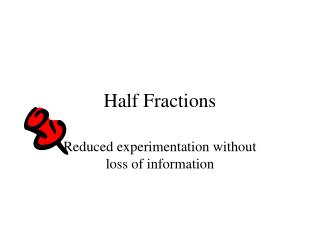DownloadDownload PresentationHalf Fractions

Half Fractions

Télécharger la présentationHalf Fractions

- - - - - - - - - - - - - - - - - - - - - - - - - - - E N D - - - - - - - - - - - - - - - - - - - - - - - - - - -
Presentation Transcript

1. Half Fractions Reduced experimentation without loss of information

2. 16*15*14*13*12*11*10*9 16 = 8 8*7*6*5*4*3*2*1 = 12870 24-1 Factorial Design Choose 8 experiments out of 16 The number of possible combinations:

3. Optimal Resolution • Full Factorial design for the k-1 first variables. • The Contrast coefficient for variable k is defined as :  x1 x2.. xk-1

4. X3 X3 X1 X2 X2 X1 Why use half fractions If one of the variables are not significant in such a 23-1 FD, the design will be full for the two other variables.

5. [xi]2 = [I] [xi] [xj] = [xj] [xi] The 24-1 half fraction Generator: [x4] = [x1] [x2] [x3] Definition relation: [I] = [x1] [x2] [x3] [x4] The confounding pattern is decided from the definition relation and the following properties:

6. Design Matrix for Optimal Resolution 24-1-design, [x4] = [x1] [x2] [x3]

7. The effects for the half fraction

8. Normal plot of the regression coefficients

9. A bar diagram of the regression coefficients

10. The model ? y = 72.125 - 4.375*x1 + 11.875*x2 - 3.125*x4 + 2.625*x1x3/x2x4 ?

11. Complementary half fraction Generator: [x4] = -[x1] [x2] [x3] Exp. -234 -134 -124 -123 -34 -24 -23 -1234 No. 1 2 3 4 12 13 14 mean Yield 9 -1 -1 -1 1 1 1 -1 1 61 2 1 -1 -1 -1 -1 -1 -1 1 61 3 -1 1 -1 -1 -1 1 1 1 90 12 1 1 -1 1 1 -1 1 1 83 5 -1 -1 1 -1 1 -1 1 1 68 14 1 -1 1 1 -1 1 1 1 51 15 -1 1 1 1 -1 -1 -1 1 85 8 1 1 1 -1 1 1 -1 1 80

12. The effects for the new half fraction

13. The final model Yield, Y = 72.250 - 4.000*x1 + 12.000*x2 - 2.750*x4 + 2.250*x2x4

14. Conclusion First order model with 4 input variables: 5 parameters to decide 24Factorial Design  16 Experiments 24-1Half Fraction  8 Experiments A half fraction will be sufficient for estimating 5 parameters